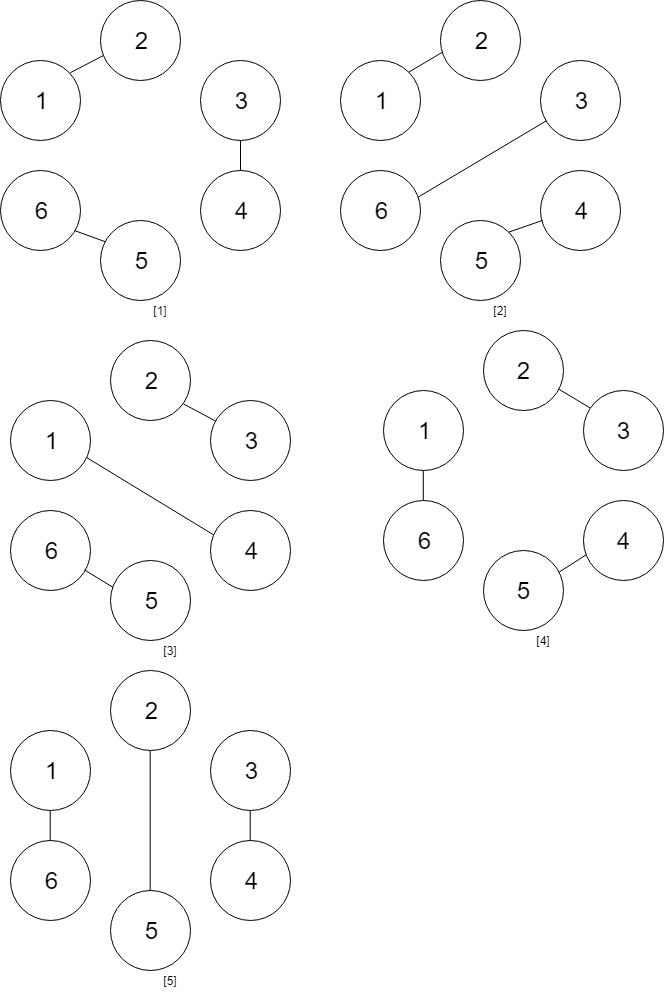1259. Handshakes That Don't Cross

You are given an even number of people `num_people` that stand around a circle and each person shakes hands with someone else, so that there are `num_people / 2` handshakes total.

Return the number of ways these handshakes could occur such that none of the handshakes cross.

Since this number could be very big, return the answer mod `10^9 + 7`

Example 1:

```Input: num_people = 2
Output: 1
```

Example 2:```Input: num_people = 4
Output: 2
Explanation: There are two ways to do it, the first way is [(1,2),(3,4)] and the second one is [(2,3),(4,1)].
```

Example 3:```Input: num_people = 6
Output: 5
```

Example 4:

```Input: num_people = 8
Output: 14
```

Constraints:

• `2 <= num_people <= 1000`
• `num_people % 2 == 0`

1259. Handshakes That Don't Cross
``````struct Solution;
use std::collections::HashMap;

const MOD: i64 = 1_000_000_007;

impl Solution {
fn number_of_ways(num_people: i32) -> i32 {
let mut memo: HashMap<i32, i64> = HashMap::new();
memo.insert(2, 2);
memo.insert(1, 1);
memo.insert(0, 1);
Self::dp(num_people / 2, &mut memo) as i32
}

fn dp(n: i32, memo: &mut HashMap<i32, i64>) -> i64 {
if let Some(&res) = memo.get(&n) {
return res;
}
let mut res = 0;
for i in 0..n {
res += Self::dp(i, memo) * Self::dp(n - 1 - i, memo);
res %= MOD;
}
memo.insert(n, res);
res
}
}

#[test]
fn test() {
let num_people = 6;
let res = 5;
assert_eq!(Solution::number_of_ways(num_people), res);
let num_people = 140;
let res = 685542858;
assert_eq!(Solution::number_of_ways(num_people), res);
}
``````